# The distribution of primes

There are many interesting questions concerning the statistical properties of the distribution of the primes. One would like to know such things as the distribution of gaps between primes, statistics for the number of primes in various sized intervals, etc.

All of those questions appear to be far out of reach (in terms of proving them), but for some of them we may be close to identifying the connection with the zeros of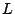-functions. For example, Goldston and Montgomery [ MR 90h:11084] prove an equivalence between the pair correlation conjecture for the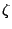-function and a statement about the variation of the distribution of primes in short intervals. Farmer and Gonek, in work in progress, extend this result to the case of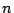-correlation, and this time the equivalence is with the variation of the distribution of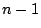almost-primes in short intervals. These results suggest that it will require something beyond the correlation functions (or more than just the-function) in order to obtain more precise results about the distribution of primes.

The Cramèr model for the distribution of primes asserts that the primes are independently distributed and the gaps between primes obey Poisson statistics. In particular,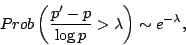for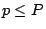, as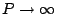. And more generally,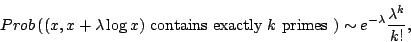for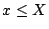, as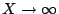Gallagher [MR 53 #13140] showed that the above prime gap distribution follows from a version of the Hardy-Littlewood conjectures.

The Cramér model is only accurate for very crude measurements of the distribution of primes. For example, Goldston and Montgomery [ MR 90h:11084] have shown that the pair correlation conjecture is equivalent to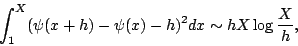for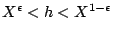. Montgomery and Soundararajan [ arXiv:math/0003234] note that the above formula can be interpreted as suggesting that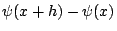is normally distributed with mean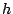and variance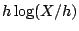, for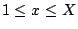and, while the Cramér model predicts meanand variance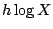. They then use numerical and heuristic evidence to develop a more sophisticated probabalistic model for the distribution of primes in short intervals.

Back to the main index for L-functions and Random Matrix Theory.